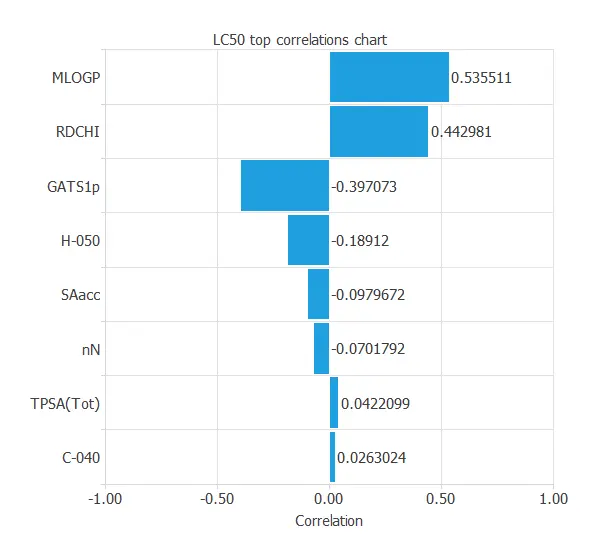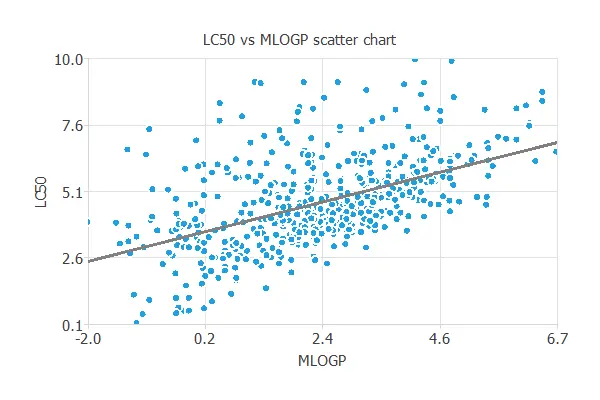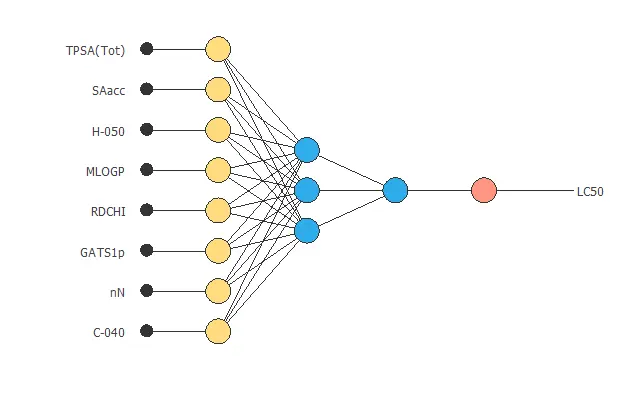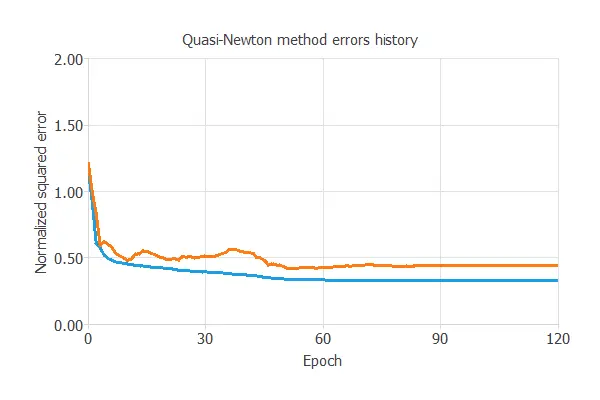﻿ Aquatic toxicity modeling using machine learning

# Modeling the toxicity of a waterbody using neural networks

Many chemicals partition in water and can exert adverse effects on aquatic systems threatening the survival of the members of these ecosystems.

Calculating a standard measure of aquatic toxicity is a long and costly procedure. However, using actual data from a waterbody, we can model this variable using Machine Learning techniques. As a result, we do not need to measure it in the laboratory.### Contents:

This example is solved with Neural Designer. You can use the free trial to understand how the solution is achieved step by step.

## 1. Application type

This is an approximation project since the variable to be predicted is continuous.

The fundamental goal here is to model the LC50 (the standard measure of toxicity) as a function of the sample's molecular properties.

## 2. Data set

The first step is to prepare the data set . This is the source of information for the approximation problem. It is composed of:

• Data source.
• Variables.
• Instances.

The file aquatic-toxicity.csv contains the data for this example. Here the number of variables (columns) is 9, and the number of instances (rows) is 546.

We have the following variables for this analysis:

• TPSA, represents the topological polar surface area calculated by means of a contribution method that takes into account N, O, P and S.
• SAacc , describes the Van der Waals surface area (VSA) of atoms that are acceptors of hydrogen bonds.
• H-050, represents the number of hydrogen atoms bonded to heteroatoms.
• MLOGP , is the octanol–water partition coefficient (LogP) calculated from the Moriguchi model.
• RDCHI , is a topological index that encodes information about molecular size and branching, but does not account for heteroatoms.
• GATS1p , encodes information on molecular polarisability.
• nN , is the number of nitrogen atoms present in the molecule.
• C-040 , represents the number of carbon atoms of the type R–C(=X)–X / R–C#X / X=C=X, where X represents any electronegative atom (O, N, S, P, Se, halogens).
• LC50 , standard toxicity measure (mean lethal concentration) (-Log(mol/L)).

Our target variable will be the last one, LC50.

The instances are divided into training, selection, and testing subsets. They represent 60%, 20%, and 20% of the original instances, respectively, and are split at random.

Calculating the data distributions helps us check for the correctness of the available information and detect anomalies. The following chart shows the histogram for the power-generated variable:It is also interesting to look for dependencies between input and target variables. To do that, we can plot an inputs-targets correlations chart.MLOGP and RDCHI are the most correlated variables because they measure lipophilicity, which is the driving force of narcosis.

In a scatter chart we can visualize how this correlation works.### 3. Neural network

The second step is to build a neural network that represents the approximation function. For approximation problems, it is usually composed by:

• Scaling layer.
• Perceptron layers.
• Unscaling layer.

The neural network has 8 inputs (TPSA, SAacc, H-050, MLOGP, RDCHI, GATS1p, nN, C-040) and 1 output (LC50).

The scaling layer contains the statistics of the inputs. We use the automatic setting for this layer to accommodate the best scaling technique for our data.

We use 2 perceptron layers here:

The unscaling layer contains the statistics of the outputs. We use the automatic method as before.

The next graph represents the neural network for this example.## 4. Training strategy

The fourth step is to select an appropriate training strategy. It is composed of two parameters:

• Loss index.
• Optimization algorithm.

The loss index defines what the neural network will learn. It is composed of an error term and a regularization term .

The error term chosen is the normalized squared error . It divides the squared error between the outputs from the neural network and the targets in the data set by its normalization coefficient. If the normalized squared error has a value of 1, then the neural network is predicting the data 'in the mean,' while a value of zero means a perfect prediction of the data. This error term does not have any parameters to set.

The regularization term is the L2 regularization . It is applied to control the complexity of the neural network by reducing the value of the parameters. We use a weak weight for this regularization term.

The optimization algorithm is in charge of searching for the neural network parameters that minimize the loss index. Here we chose the quasi-Newton method as optimization algorithm.The following chart shows how the training (blue) and selection (orange) errors decrease with the epochs during the training process. The final values are training error = 0.331 NSE and selection error = 0.481 NSE , respectively.

Even though we are getting moderately good results, our model is far from perfect, mainly because of the small size of the Data Set we are working with. This might be one of the biggest issues of Machine Learning.

## 5. Model selection

In this case, Model selection algorithms aren't very useful to improve our model's performance, as having a more complex arquitecture can also broaden the small Data Set problem.

## 6. Testing analysis

The purpose of the testing analysis is to validate the generalization capabilities of the neural network. We use the testing instances in the data set, which have never been used before.

A standard testing method in approximation applications is to perform a linear regression analysis between the predicted and the real pollutant level values.For a perfect fit, the correlation coefficient R2 would be 1. As we have R2 = 0.744, the neural network is predicting the testing data quite well, considering our small Data Set issues.

We have achieved a mean error of 8.64%.

## 7. Model deployment

In the model deployment phase, the neural network is used to predict outputs for inputs that it has never seen.

We can calculate the neural network outputs for a given set of inputs:

• TPSA(Tot): 48.473.
• SAacc: 58.869.
• H-050: 0.938.
• MLOGP: 2.313.
• RDCHI: 2.492.
• GATS1p: 1.046.
• nN: 1.004.
• C-040: 0.3534.
• LC50: 4.92.

For that purpose, we can use Response Optimization . The objective of the response optimization algorithm is to exploit the mathematical model to look for optimal operating conditions.
Indeed, the predictive model allows us to simulate different operating scenarios and adjust the control variables to improve efficiency.

An example is to minimize LC50 toxicity while maintaining the number of nitrogen atoms equal to the desired value.

The next table resumes the conditions for this problem.

Variable name Condition
TPSA(Tot) None
SAacc None
H-050 None
MLOGP None
RDCHI None
GATS1p None
nN Equal to 2
C-040 None
LC50 Minimize

The next list shows the optimum values for previous conditions.

• TPSA(Tot): 93.6666.
• SAacc: 345.496.
• H-050: 1.17883.
• MLOGP: -4.5905.
• RDCHI: 4.53847.
• GATS1p: 1.30937.
• nN: 2.
• C-040: 6.24457.
• LC50: 0.18981.

Directional outputs plot the neural network outputs through some reference points.

The next list shows the reference point for the plots.

• TPSA(Tot): 48.473.
• SAacc: 58.869.
• H-050: 0.938.
• MLOGP: 2.313.
• RDCHI: 2.492.
• GATS1p: 1.046.
• nN: 1.004.
• C-040: 0.3534.

We can see here how MLOGP affects LC50:The mathematical expression represented by the predictive model is displayed next:

`            scaled_TPSA(Tot) = TPSA(Tot)*(1+1)/(347.3200073-(0))-0*(1+1)/(347.3200073-0)-1;scaled_SAacc = SAacc*(1+1)/(571.9520264-(0))-0*(1+1)/(571.9520264-0)-1;scaled_H-050 = H-050*(1+1)/(18-(0))-0*(1+1)/(18-0)-1;scaled_MLOGP = MLOGP*(1+1)/(9.147999763-(-6.446000099))+6.446000099*(1+1)/(9.147999763+6.446000099)-1;scaled_RDCHI = RDCHI*(1+1)/(6.43900013-(1))-1*(1+1)/(6.43900013-1)-1;scaled_GATS1p = GATS1p*(1+1)/(2.5-(0.2809999883))-0.2809999883*(1+1)/(2.5-0.2809999883)-1;scaled_nN = nN*(1+1)/(11-(0))-0*(1+1)/(11-0)-1;scaled_C-040 = C-040*(1+1)/(11-(0))-0*(1+1)/(11-0)-1;perceptron_layer_output_0 = tanh[ 0.291703 + (scaled_TPSA(Tot)*-0.391549)+ (scaled_SAacc*0.251752)+ (scaled_H-050*0.085857)+ (scaled_MLOGP*-0.277858)+ (scaled_RDCHI*-1.14629)+ (scaled_GATS1p*0.803457)+ (scaled_nN*-0.240904)+ (scaled_C-040*-0.137404) ];perceptron_layer_output_1 = tanh[ 0.240456 + (scaled_TPSA(Tot)*0.895344)+ (scaled_SAacc*-0.575449)+ (scaled_H-050*0.216825)+ (scaled_MLOGP*0.676507)+ (scaled_RDCHI*0.277635)+ (scaled_GATS1p*-0.115849)+ (scaled_nN*-0.0410888)+ (scaled_C-040*-0.239895) ];perceptron_layer_output_2 = tanh[ 1.35559 + (scaled_TPSA(Tot)*0.868684)+ (scaled_SAacc*-0.00789895)+ (scaled_H-050*0.878771)+ (scaled_MLOGP*0.816033)+ (scaled_RDCHI*-2.5133)+ (scaled_GATS1p*2.02157)+ (scaled_nN*-0.148986)+ (scaled_C-040*-1.02231) ];perceptron_layer_output_0 = [ -0.309113 + (perceptron_layer_output_0*2.07224)+ (perceptron_layer_output_1*2.20069)+ (perceptron_layer_output_2*-1.55368) ];unscaling_layer_output_0 = perceptron_layer_output_0*(10.04699993-0.1220000014)/(1+1)+0.1220000014+1*(10.04699993-0.1220000014)/(1+1);        `

## References:

• M. Cassotti, D. Ballabio, V. Consonni, A. Mauri, I. V. Tetko, R. Todeschini (2014). Prediction of acute aquatic toxicity towards daphnia magna using GA-kNN method, Alternatives to Laboratory Animals (ATLA), 42,31:41; doi: 10.1177/026119291404200106